# Absolute neighbourhood extensor

Jump to: navigation, search

ANE

For the time being, assume that all topological spaces under discussion are metrizable (cf. also Metrizable space). A spaceis called an absolute (neighbourhood) extensor, abbreviated AE (respectively, ANE), provided that for every space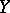and every closed subspace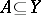, every continuous function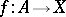can be extended over(respectively, over a neighbourhood ofin). The classical Tietze extension theorem (cf. also Extension theorems) implies that familiar spaces such as the real line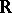, the unit intervaland the circle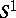are absolute (neighbourhood) extensors. An absolute (neighbourhood) retract is a spacehaving the property that wheneveris embedded as a closed subset of a space, then it is a (neighbourhood) retract of(cf. also Absolute retract for normal spaces; Retract of a topological space). It is a fundamental theorem that every AE (respectively, ANE) is an AR (respectively, ANR), and conversely. The theory of absolute (neighbourhood) retracts was initiated by K. Borsuk in [a1], [a2]. He proved his fundamental homotopy extension theorem in [a3]: Ifis a closed subspace of a spaceandis an ANR and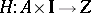is a homotopy such thatis extendable to a function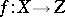, then there is a homotopy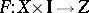such that, and for every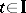,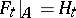. For more details, see [a4] and [a10].

In 1951, J. Dugundji [a9] proved that a convex set in a locally convex vector space (cf. also Locally convex space) is an AR. This result was a major improvement over the Tietze extension theorem and was widely applied. The fundamental problem whether the local convexity assumption in this result could be dropped, was solved by R. Cauty [a5] in the negative. His counterexample used in an essential way a theorem of A.N. Dranishnikov [a8] about the existence of an infinite-dimensional compactum with finite cohomological dimension.

There are several topological characterizations of absolute (neighbourhood) retracts. The most useful one is due to S. Lefschetz [a11] and is in terms of partial realizations of polytopes in the space under consideration. It can be shown that if a spaceis dominated (cf. also Homotopy type) by a simplicial complex, then it has the homotopy type of another simplicial complex (see [a12]). Since it is not too hard to prove that every ANR is dominated by a simplicial complex (see [a10]), it follows, in particular, that every ANR has the homotopy type of some simplicial complex. But the natural question whether every compact ANR has the homotopy type of a compact simplicial complex, i.e. a finite polyhedron, remained unanswered for a long time. It was finally solved in the affirmative by J.E. West [a17] by using powerful results from T.A. Chapman [a6] in infinite-dimensional topology.

Another fundamental problem about absolute (neighbourhood) retracts was Borsuk's problem of whether for compact absolute (neighbourhood) retractsand, the topological dimension of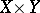is equal to the sum of the dimensions ofand, respectively. This problem was solved by Dranishnikov [a7], who proved that there exist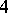-dimensional compact absolute retractsandwhose product is of dimension.

The theory of absolute (neighbourhood) retracts played a key role in infinite-dimensional topology. The fundamental topological characterization results of manifolds over the Hilbert cube and the Hilbert space, respectively, which are due to H. Toruńczyk [a15], [a16], are stated in terms of absolute (neighbourhood) retracts. Many of the remaining open problems in infinite-dimensional topology have been proven to actually be problems about absolute (neighbourhood) retracts.

The theory of absolute (neighbourhood) retracts in Tikhonov spaces (cf. also Tikhonov space). was mainly considered by E.V. Shchepin. He proved in [a13] that finite-dimensional compact absolute (neighbourhood) retracts are metrizable. He also found, in [a14], a very interesting topological characterization of all Tikhonov cubes of uncountable weight. ANR-theory plays a crucial role in this characterization.